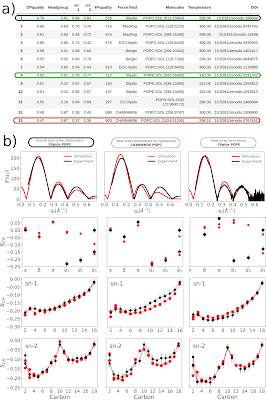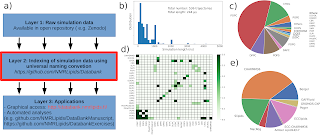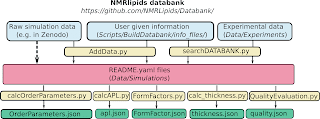## Wednesday, May 18, 2022

### NMRlipids databank: Quality evaluation

Defining a quantitative quality measure for lipid bilayer simulations has been one of the goals of the NMRlipids project since the beginning. Such measure is highly useful when selecting the best force field for a specific application, and for improving force field parameters, particularly with automated procedures. Based on literature review and results of the NMRlipids Project, summarized in the NMRlipids V publication, we have concluded that the C-H bond order parameters from NMR can be used to evaluate the conformational ensembles of individual lipids, and the x-ray scattering form factors can be used to evaluate the lipid bilayer dimensions. Based on the work in the NMRlipids workshops in Berlin (2019) and Prague (2021), we have now written a code that evaluates the quality of simulations in the NMRlipids Databank. The key ideas and results of the quality evaluation are described in this post. More details and results can be found from the NMRlipids Databank manuscript and from GitHub.

Results. The order parameter quality of 58 simulations and the form factor quality of 99 simulations have so far been evaluated in the NMRlipids Databank. Figure 1a shows the results for the 13 best simulations according to the overall order parameter quality; and Figure 1b shows comparison between simulations and experiments for the best simulations concerning the overall order parameters (left column), the headgroup order parameters (middle column), and the form factor (right column). Results for all ranked simulations ordered in various ways are available on GitHub.Figure 1: a) The best 13 simulations currently in the NMRlipids Databank according to the overall order parameter quality. b) Comparison of x-ray scattering form factors and C-H bond order parameters between simulations and experiments demonstrated for the simulations giving the best qualities in the overall order parameters (left column), for the headgroup order parameters (middle), for the and x-ray scattering form factor (right).

Conformational ensembles evaluated against C-H bond order parameters from NMR. After the workshop in Prague, our idea was to define the poorness Š of each order parameter as Š=-log(P); here P is the probability mass within the experimental error for a normal distribution, whose mean is the order parameter from the simulation, and whose standard deviation is the standard error of the mean from the simulation. However, when testing this definition of Š on the simulations in the NMRlipids Databank, it turned out that the probability of the simulated order parameters to locate within experimental errors was often below the numerical accuracy of computers. To avoid such numerical instability, we decided to use the first order Student’s t-distribution instead, and calculate the probability from the equation\begin{equation}  P = f \left( \frac{S_{\rm CH} - (S_{\rm exp}+\Delta S_{\rm exp})}{s/\sqrt{n}} \right) - f \left( \frac{S_{\rm CH} - (S_{\rm exp}-\Delta S_{\rm exp})}{s/\sqrt{n}} \right),
\end{equation}where f(t) is the first order Student's t-distribution, s is the variance of the order parameter SCH calculated over individual lipids and n is the number of lipids in the simulation. Because Student's t-distribution has heavier tails than normal distribution, even order parameters far from experiments have distinguishable non-zero probabilities. Therefore, the logarithm used to define the poorness Š is not needed, and we report the qualities directly as probabilities. However, it should be noted that using the first order Student's t-distribution instead of the normal distribution slightly underestimates the statistical accuracy of order parameters calculated from simulations.

In order to rank simulations based on headgroup, acyl chain, or individual lipid qualities, the average probabilities can be calculated over lipid fragments and types. For more details see the NMRlipids Databank manuscript.

Lipid bilayer dimensions evaluated against x-ray scattering form factor. The qualities of form factors in simulations are evaluated as in the SIMtoEXP program \begin{equation}
\chi^2 = \frac{\sqrt{\sum_{i=1}^{N_q}(|F_s(q_i)|-k_e|F_e(q_i)|)^2/(\Delta F_e(q_i))^2}}{\sqrt{N_q-1}},
\end{equation}
where Fs is the form factor from simulation and Fe from experiment, the summation goes over the experimentally available Nq points, and \begin{equation}
k_e = \frac{\sum_{i=1}^{N_q} \frac{|F_s(q_i)||F_e(q_i)|}{(\Delta F_e(q_i))^2}}{\sum_{i=1}^{N_q} \frac{|F_e(q_i)|^2}{(\Delta F_e(q_i))^2}}.
\end{equation}It should be noted that in this evaluation the simulation uncertainty is not accounted for in any way.

## Monday, May 9, 2022

### NMRlipids databank: Current status and structure

After the latest NMRlipids publication, the main focus has been in the development of the NMRlipids databank that would enable automatic analyses over all the data contributed to the project. After the original idea and preliminary version, the development has been facilitated by several meetings (Berlin, online I, online II, Prague, online III). The upcoming meeting in Helsinki on 1.-3.6.2022 will contain also educative parts for using the NMRlipids databank.

The databank is now essentially functional and preparation of the first manuscript has been started. The overlay structure and current content of the databank are illustrated in figure 1. The structure of the databank is discussed more detailed below. Quality evaluation and preliminary results will be discussed in the upcoming posts.Figure 1: a) Structure of an overlay databank. More detailed structure of the layer 2 in the NMRlipids databank is illustrated in figure 2. b) Distribution of the lengths of the trajectories, total number of trajectories and total lenght of the simulations in the NMRlipids databank. c) Distribution of lipids present in the trajectories in the NMRlipids databank. Lipids occuring in five or less simulations (’others’) are listed in the right. d) Currently available binary mixtures in the NMRlipids databank. e) Distribution of force fields in the simulations in the NMRlipids databank. The figures and numbers are created on 9th of May 2022 with stats.ipynb.

Structure of the NMRlipids databank. As illustrated in Fig. 2., the AddData.py script creates a README.yaml file that contains all the essential information of an added simulation based on the information given according to the instructions. The created README.yaml files are stored in folders in Data/Simulations. The folders are named after the hash identities of trajectory and topology files. While the raw simulation data is not directly stored in the NMRlipids databank, the README.yaml files contain permanent links from where the raw data can be accessed when needed.

For the quality evaluation, simulations are connected to the available experimental C-H bond order parameters from NMR and x-ray scattering from factors, which are also included in the NMRlipids databank. The connection between a simulation and experimental data set is made by the SearchDATABANK.py script when molar concentrations of all molecules are within ±5 percentage units, charged lipids have the same counterions, and temperature is within ±2 degrees. In such cases, the paths to the experimental data are added into the simulation README.yaml file.Figure 2: Figure 2: Structure of the NMRlipids databank. Manually added input data (blue boxes) includes basic information on the simulation (more details from here), permanent links to the raw data, and experimental data if available. The databank entries (red box) and analysis results (green boxes) are automatically generated by the computer programs included in the NMRlipids databank (yellow boxes) and stored in here. Because raw data are not permanently stored in the NMRlipids databank but can be accessed based on the information in the databank, this connection is marked with the dashed line.

Analysing simulations in the NMRlipids databank. Because README.yaml files contain all the essential information from each simulation, including the permanent location of raw data and unique naming convention for all atoms and molecules (see below), arbitrary analyses of simulations can be automatically performed for all simulations in the NMRlipids databank. For example, the code that calculates all C-H bond order parameters of all systems first loops over all README.yaml files (i.e., simulations) in the NMRlipids databank, then downloads the raw simulation to a local computer if needed, and then uses the information about the atom and molecule naming conventions in README.yaml and mapping files to perform the desired analyses. A minimal example of an analysis code is available in here. Results for order parameters, form factors, area per lipid and thickness are stored in same locations as README.yaml files. Further analyses can be conventiently stored in separate repositories with the same folder structure based on hash identities of trajectory and topology files as done, for example, for the preparation of the NMRlipids databank manuscript.

Molecule and atom naming convention. Unique naming convetions for molecules and atoms are needed for automatic analyses over large sets of simulation data in the NMRlipids databank. Because such convention was not available for lipids, we have generated mapping files (available in here) that connect lipid atoms names in each simulation to the universal atom names and universal abbreviations of lipid names for the NMRlipids databank (see the second table in here). For a new entry into the NMRlipids databank, the universal abbreviation for each lipid and the corresponding mapping file are given as input in the COMPOSITION dictionary. The numbers of each molecule in the simulation are then automatically calculated by the AddData.py (see figure 2) and stored in the COMPOSITION dictionary in README.yaml files. This information enables selection of any molecule or atom when analysing simulations in the NMRlipids databank.